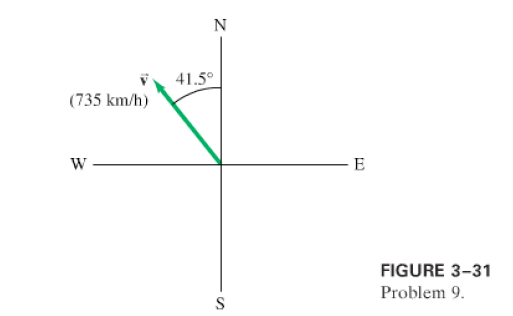×
Get Full Access to Physics: Principles With Applications - 6 Edition - Chapter 3 - Problem 9p
Get Full Access to Physics: Principles With Applications - 6 Edition - Chapter 3 - Problem 9p

×

# An airplane is traveling 735 km/h in a direction 41.5°ISBN: 9780130606204 3

## Solution for problem 9P Chapter 3

Physics: Principles with Applications | 6th Edition

• Textbook Solutions
• 2901 Step-by-step solutions solved by professors and subject experts
• Get 24/7 help from StudySoup virtual teaching assistantsPhysics: Principles with Applications | 6th Edition

4 5 1 396 Reviews
26
2
Problem 9P

(II) An airplane is travelingin a directionwest of north (Fig.). () Find the components of the velocity vector in the northerly and westerly directions.(b) How far north and how far west has the plane traveled afterStep-by-Step Solution:

Solution 9P Introduction Given in the question that 25% of chemical energy is converted to mechanical energy. Therefore, remaining (100-25)% = 75% will be mechanical energy as Total energy = Chemical energy+mechanical energy. To calculate increase in body temperature, we need to use the equation Q = ms, where Q is amount of heat involved, m is the mass of J cyclist, s is specific heat of human body = 3400 kg. C, is increase in body temperature. Step 1: Total metabolic power is = 480 W Now, 75% of total metabolic power is converted to thermal energy.

Step 2 of 4

Step 3 of 4

##### ISBN: 9780130606204

This full solution covers the following key subjects: Energy, Thermal, Excess, rid, Able. This expansive textbook survival guide covers 35 chapters, and 3914 solutions. The answer to “?(II) An airplane is traveling in a direction west of north (Fig. ). ( ) Find the components of the velocity vector in the northerly and westerly directions.(b) How far north and how far west has the plane traveled after” is broken down into a number of easy to follow steps, and 40 words. This textbook survival guide was created for the textbook: Physics: Principles with Applications, edition: 6. Since the solution to 9P from 3 chapter was answered, more than 1237 students have viewed the full step-by-step answer. The full step-by-step solution to problem: 9P from chapter: 3 was answered by , our top Physics solution expert on 03/03/17, 03:53PM. Physics: Principles with Applications was written by and is associated to the ISBN: 9780130606204.

Unlock Textbook Solution